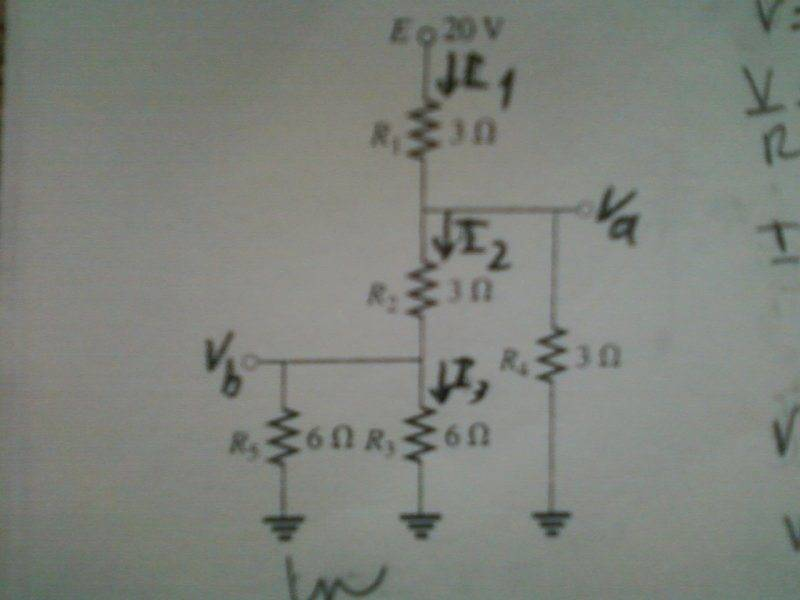# Understanding how to re-draw circuits

• Engineering
• Lopez
In summary, The conversation discusses how to find the values of I1, I2, I3, Va, and Vb in a complex circuit. The individual is struggling to understand how to read the circuit and identify which components are in series or parallel. They suggest simplifying the circuit by combining resistors and using Kirchhoff's Current Law (KCL) to find the unknown values. They also mention the importance of maintaining the individual voltage and current of each component. The conversation ends with a discussion on how to solve for Vb if a certain resistor is removed from the circuit.

## Homework Statement

I need to find I1,I2,I3,Va,Vb but i don't even know to read the circuit. i know how to to do series circuits and parallel circuits but not this. How do i read this circuit and know which ones are in parallel or in series. is there a way to re-draw it? I have the solution and answer. Therefore i don't need the step by step solution since i already have it,is just that it doesn't explain why they did it that why.
These are the I1=4A ,I2=1.33, I3=.67, I4=2.67,va=8.0,and vb i don't know.Last edited:
If you need a loop, put in a 20 V battery with the positive plate connected to E. Connect the negative plate of the battery to the 3 grounds at the bottom of the diagram.

•Lopez
I would work my way up from the bottom, simplifying bits and pieces as I go. R5 and R3 are in parallel, right? So you could combine those two resistors into a single resistance. Keep chipping away at it until you have a single loop.

•Lopez
If you combine circuit elements you will lose that components individual voltage and/or current.

I reccoend doing a KCL at Va and Va. Then solve for Va and Vb, and the currents will be easy to find.

thanks for the tip

Mark44 said:
I would work my way up from the bottom, simplifying bits and pieces as I go. R5 and R3 are in parallel, right? So you could combine those two resistors into a single resistance. Keep chipping away at it until you have a single loop.
if i do ((R5||R3)+R2)||R4 and this would be in series with R1 right? now to get Vb i think i would do Vb=R5(I2-I3) but what if that R5 was not there then how would i solve for vb

Lopez said:
if i do ((R5||R3)+R2)||R4 and this would be in series with R1 right? now to get Vb i think i would do Vb=R5(I2-I3) but what if that R5 was not there then how would i solve for vb
If R5 was not there then I2 and I3 would change. you cannot solve for an unknown value with unknown values